• 前两天做数据结构实验，要求用非递归算法遍历二叉树。只知道用栈来储存数据，具体算法还不太清楚。经过两天的搜索，看到网上很多种解法，很多解法都是用C++来写的算法，一直找不到用C语言写的算法，所以就总结了一下...
前两天做数据结构实验，要求用非递归算法遍历二叉树。只知道用栈来储存数据，具体算法还不太清楚。经过两天的搜索，看到网上很多种解法，很多解法都是用C++来写的算法，一直找不到用C语言写的算法，所以就总结了一下，用C写出了一个遍历二叉树的三种非递归算法。
前序遍历
前序遍历按照“根结点-左孩子-右孩子”的顺序进行访问。 具体算法：先遍历左孩子，并输出。当左孩子遍历完后，取栈顶，找右孩子。此时循环还没有结束，再遍历它的左孩子，右孩子直至孩子全部遍历结束。
void preorder(bitree *t)//前序遍历的非递归算法
{
bitree *temp = t;//定义一个树节点，用它来遍历
while(temp != NULL || s.top != 0)
{
while(temp != NULL)//先遍历左孩子，并输出。
{
printf("%4d",temp->data);
push(temp);
temp = temp->lchild;
}
if(s.top != 0)//当左孩子遍历完后，取栈顶，找右孩子。此时循环还没有结束，再遍历它的左孩子，直至孩子全部遍历结束。
{
temp = pop();
temp = temp->rchild;
}
}
printf("\n");
}

中序遍历
中序遍历按照“左孩子-根节点-右孩子”的顺序进行访问。 具体算法：先把左孩子入栈，不着急先输出，先把所有左孩子入栈。左孩子入栈结束，取栈顶，输出栈顶元素，遍历右孩子，右孩子入栈。
void inorder(bitree *t)//中序遍历的非递归算法
{
bitree *temp = t;
while(temp != NULL||s.top != 0)
{
while(temp != NULL)//先把左孩子入栈，所有左孩子入栈结束
{
push(temp);
temp = temp->lchild;
}
if(s.top != 0)//左孩子入栈结束，取栈顶，输出栈顶元素，遍历右孩子
{
temp = pop();
printf("%4d",temp->data);
temp = temp->rchild;
}
}
printf("\n");
}

后序遍历
后序遍历按照“左孩子-右孩子-根节点”的顺序进行访问。 具体算法：对于任一结点P，将其入栈，然后沿其左子树一直往下搜索，直到搜索到没有左孩子的结点，此时该结点出现在栈顶，但是此时不能将其出栈并访问，因此其右孩子还为被访问。所以接下来按照相同的规则对其右子树进行相同的处理，当访问完其右孩子时，该结点又出现在栈顶，此时可以将其出栈并访问。这样就保证了正确的访问顺序。可以看出，在这个过程中，每个结点都两次出现在栈顶，只有在第二次出现在栈顶时，才能访问它。因此需要多设置一个变量标识该结点是否是第一次出现在栈顶。
void laorder(bitree *root)//后序遍历的非递归算法
{
bitree *temp = root;
while(temp!=NULL||s.top!=0)
{
while(temp!= NULL)
{
temp->cishu=1;       // 当前节点首次被访问
push(temp);
temp=temp->lchild;
}
if(s.top!=0)
{
temp=pop( );
if(temp->cishu == 1)   // 第一次出现在栈顶
{

temp->cishu++;
push(temp);
temp=temp->rchild;
}
else
if(temp->cishu==2)//第二次输出并制空，防止陷入死循环
{
printf("%4d",temp->data);
temp=NULL;
}
}
}
printf("\n");
}

一个完整的程序
#include <stdio.h>
#include <stdlib.h>
#define NULL 0
#define M 100
typedef struct node
{
int data;
int cishu;
struct node *lchild;
struct node *rchild;  //树节点中cishu是为了计数用。在后序遍历中，子树的根节点在第一次遍历的时候不会输出，只有在第二次遍历的时候才输出。
}bitree;

typedef struct stack
{
bitree *elements[M];
int top;
}seqstack;//定义一个储存树类型地址的栈，方便遍历的时候追踪到树的地址。

bitree *root;//定义一个树根
seqstack s;//定义栈

void setnull()//初始化栈
{
s.top =0;
}

void push(bitree *temp)//入栈操作
{
s.elements[s.top++] = temp;
}

bitree *pop()//取栈顶并出栈顶
{
return s.elements[--s.top];
}

int empty()//判断空栈
{
return s.top == 0;
}

bitree *creat()   /*建立二叉树的递归算法*/
{ bitree *t;
int x;
scanf("%d",&x);
if(x==0) t=NULL; /*以x=0表示输入结束*/
else{
t=(bitree*)malloc(sizeof(bitree));//动态生成结点t,分别给结点t的数据域、左右孩子域
t->data=x;                  //赋值,给左右孩子域赋值时用到了递归的思想。
t->lchild=creat();
t->rchild=creat();
}
return t;
}

void preorder(bitree *t)//前序遍历的非递归算法
{
bitree *temp = t;//定义一个树节点，用它来遍历
while(temp != NULL || s.top != 0)
{
while(temp != NULL)//先遍历左孩子，并输出。
{
printf("%4d",temp->data);
push(temp);
temp = temp->lchild;
}
if(s.top != 0)//当左孩子遍历完后，取栈顶，找右孩子。此时循环还没有结束，再遍历它的左孩子，直至孩子全部遍历结束。
{
temp = pop();
temp = temp->rchild;
}
}
printf("\n");
}

void inorder(bitree *t)//中序遍历的非递归算法
{
bitree *temp = t;
while(temp != NULL||s.top != 0)
{
while(temp != NULL)//先把左孩子入栈，所有左孩子入栈结束
{
push(temp);
temp = temp->lchild;
}
if(s.top != 0)//左孩子入栈结束，取栈顶，输出栈顶元素，遍历右孩子
{
temp = pop();
printf("%4d",temp->data);
temp = temp->rchild;
}
}
printf("\n");
}

void laorder(bitree *root)//后序遍历的非递归算法
{
bitree *temp = root;
while(temp!=NULL||s.top!=0)
{
while(temp!= NULL)
{
temp->cishu=1;       // 当前节点首次被访问
push(temp);
temp=temp->lchild;
}
if(s.top!=0)
{
temp=pop( );
if(temp->cishu == 1)   // 第一次出现在栈顶
{

temp->cishu++;
push(temp);
temp=temp->rchild;
}
else
if(temp->cishu==2)//第二次输出并制空，防止陷入死循环
{
printf("%4d",temp->data);
temp=NULL;
}
}
}
printf("\n");
}

int main()
{
bitree *root;//创建根
setnull();//制空栈
root=creat();//创建二叉树:尝试输入：1 2 3 0 0 4 0 0 5 6 0 0 7 0 0
printf("前序遍历:\n");
preorder(root);
printf("中序遍历:\n");
inorder(root);
printf("后序遍历:\n");
laorder(root);
return 0;
}

程序验证
建立一个如下图所示的二叉树（图画的不太好看）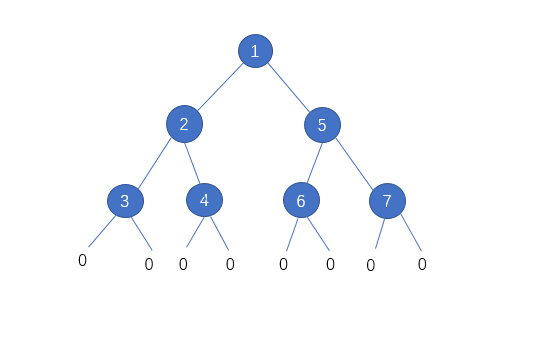结果如下图：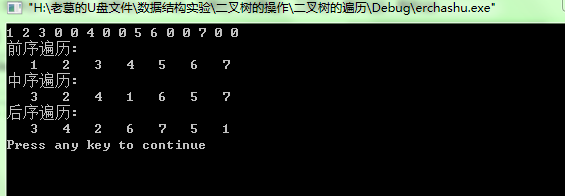展开全文二叉树 数据结构
• 最近学习二叉树，想编程实现递归和非递归的实现方式； 递归的方式就不说了，因为大家的递归程序都一样；但是对于非递归的实现方式， 根据这几天的查阅资料已看到差不多近10种不同的遍历二叉树的非递归实现方式，...
本文并非我所写，是复制的该链接中的内容；
最近学习二叉树，想编程实现递归和非递归的实现方式；
递归的方式就不说了，因为大家的递归程序都一样；但是对于非递归的实现方式，
根据这几天的查阅资料已看到差不多近10种不同的遍历二叉树的非递归实现方式，其中本文是个人觉得在前中后序三种遍历方式中，代码最统一的一种方法；
1：本文链接源于：https://www.jianshu.com/p/49c8cfd07410
2：在这里向大家推荐另外一种也是很强大的统一的实现方式，实现过程真的挺强大的，当然这也是别人的，我只是在这里总结一下，方便大家学习，该链接为：http://drinkjava2.iteye.com/blog/2353983

上面第一个链接中的内容如下：
解决二叉树的很多问题的方案都是基于对二叉树的遍历。遍历二叉树的前序，中序，后序三大方法算是计算机科班学生必写代码了。其递归遍历是人人都能信手拈来，可是在手生时写出非递归遍历恐非易事。正因为并非易事，所以网上出现无数的介绍二叉树非递归遍历方法的文章。可是大家需要的真是那些非递归遍历代码和讲述吗？代码早在学数据结构时就看懂了，理解了，可为什么我们一而再再而三地忘记非递归遍历方法，却始终记住了递归遍历方法？
三种递归遍历对遍历的描述，思路非常简洁，最重要的是三种方法完全统一，大大减轻了我们理解的负担。而我们常接触到那三种非递归遍历方法，除了都使用栈，具体实现各有差异，导致了理解的模糊。本文给出了一种统一的三大非递归遍历的实现思想。
三种递归遍历

//前序遍历
void preorder(TreeNode *root, vector<int> &path)
{
if(root != NULL)
{
path.push_back(root->val);
preorder(root->left, path);
preorder(root->right, path);
}
}


//中序遍历
void inorder(TreeNode *root, vector<int> &path)
{
if(root != NULL)
{
inorder(root->left, path);
path.push_back(root->val);
inorder(root->right, path);
}
}


//后续遍历
void postorder(TreeNode *root, vector<int> &path)
{
if(root != NULL)
{
postorder(root->left, path);
postorder(root->right, path);
path.push_back(root->val);
}
}

由上可见，递归的算法实现思路和代码风格非常统一，关于“递归”的理解可见我的《人脑理解递归》。
教科书上的非递归遍历

//非递归前序遍历
void preorderTraversal(TreeNode *root, vector<int> &path)
{
stack<TreeNode *> s;
TreeNode *p = root;
while(p != NULL || !s.empty())
{
while(p != NULL)
{
path.push_back(p->val);
s.push(p);
p = p->left;
}
if(!s.empty())
{
p = s.top();
s.pop();
p = p->right;
}
}
}


//非递归中序遍历
void inorderTraversal(TreeNode *root, vector<int> &path)
{
stack<TreeNode *> s;
TreeNode *p = root;
while(p != NULL || !s.empty())
{
while(p != NULL)
{
s.push(p);
p = p->left;
}
if(!s.empty())
{
p = s.top();
path.push_back(p->val);
s.pop();
p = p->right;
}
}
}


//非递归后序遍历-迭代
void postorderTraversal(TreeNode *root, vector<int> &path)
{
stack<TempNode *> s;
TreeNode *p = root;
TempNode *temp;
while(p != NULL || !s.empty())
{
while(p != NULL) //沿左子树一直往下搜索，直至出现没有左子树的结点
{
TreeNode *tempNode = new TreeNode;
tempNode->btnode = p;
tempNode->isFirst = true;
s.push(tempNode);
p = p->left;
}
if(!s.empty())
{
temp = s.top();
s.pop();
if(temp->isFirst == true)   //表示是第一次出现在栈顶
{
temp->isFirst = false;
s.push(temp);
p = temp->btnode->right;
}
else  //第二次出现在栈顶
{
path.push_back(temp->btnode->val);
p = NULL;
}
}
}
}

看了上面教科书的三种非递归遍历方法，不难发现，后序遍历的实现的复杂程度明显高于前序遍历和中序遍历，前序遍历和中序遍历看似实现风格一样，但是实际上前者是在指针迭代时访问结点值，后者是在栈顶访问结点值，实现思路也是有本质区别的。而这三种方法最大的缺点就是都使用嵌套循环，大大增加了理解的复杂度。
更简单的非递归遍历二叉树的方法
这里我给出统一的实现思路和代码风格的方法，完成对二叉树的三种非递归遍历。

//更简单的非递归前序遍历
void preorderTraversalNew(TreeNode *root, vector<int> &path)
{
stack< pair<TreeNode *, bool> > s;
s.push(make_pair(root, false));
bool visited;
while(!s.empty())
{
root = s.top().first;
visited = s.top().second;
s.pop();
if(root == NULL)
continue;
if(visited)
{
path.push_back(root->val);
}
else
{
s.push(make_pair(root->right, false));
s.push(make_pair(root->left, false));
s.push(make_pair(root, true));
}
}
}


//更简单的非递归中序遍历
void inorderTraversalNew(TreeNode *root, vector<int> &path)
{
stack< pair<TreeNode *, bool> > s;
s.push(make_pair(root, false));
bool visited;
while(!s.empty())
{
root = s.top().first;
visited = s.top().second;
s.pop();
if(root == NULL)
continue;
if(visited)
{
path.push_back(root->val);
}
else
{
s.push(make_pair(root->right, false));
s.push(make_pair(root, true));
s.push(make_pair(root->left, false));
}
}
}


//更简单的非递归后序遍历
void postorderTraversalNew(TreeNode *root, vector<int> &path)
{
stack< pair<TreeNode *, bool> > s;
s.push(make_pair(root, false));
bool visited;
while(!s.empty())
{
root = s.top().first;
visited = s.top().second;
s.pop();
if(root == NULL)
continue;
if(visited)
{
path.push_back(root->val);
}
else
{
s.push(make_pair(root, true));
s.push(make_pair(root->right, false));
s.push(make_pair(root->left, false));
}
}
}

以上三种遍历实现代码行数一模一样，如同递归遍历一样，只有三行核心代码的先后顺序有区别。为什么能产生这样的效果？下面我将会介绍。
有重合元素的局部有序一定能导致整体有序
这就是我得以统一三种更简单的非递归遍历方法的基本思想：有重合元素的局部有序一定能导致整体有序。 如下这段序列，局部2 3 4和局部1 2 3都是有序的，但是不能由此保证整体有序。Image Title

而下面这段序列，局部2 3 4,4 5 6,6 8 10都是有序的，而且相邻局部都有一个重合元素，所以保证了序列整体也是有序的。Image Title

应用于二叉树
基于这种思想，我就构思三种非递归遍历的统一思想：不管是前序，中序，后序，只要我能保证对每个结点而言，该结点，其左子结点，其右子结点都满足以前序/中序/后序的访问顺序,整个二叉树的这种三结点局部有序一定能保证整体以前序/中序/后序访问，因为相邻的局部必有重合的结点，即一个局部的“根”结点是另外一个局部的“子”结点。
如下图，对二叉树而言，将每个框内结点集都看做一个局部，那么局部有A,A B C,B D E,D,E,C F,F,并且可以发现每个结点元素都是相邻的两个局部的重合结点。发觉这个是非常关键的，因为知道了重合结点，就可以对一个局部排好序后，通过取出一个重合结点过渡到与之相邻的局部进行新的局部排序。我们可以用栈来保证局部的顺序（排在顺序前面的后入栈，排在后面的先入栈，保证这个局部元素出栈的顺序一定正确），然后通过栈顶元素(重合元素)过渡到对新局部的排序，对新局部的排序会导致该重合结点再次入栈，所以当栈顶出现已完成过渡使命的结点时，就可以彻底出栈输出了（而这个输出可以保证该结点在它过渡的那个局部一定就是排在最前面的），而新栈顶元素将会继续完成新局部的过渡。当所有结点都完成了过渡使命时，就全部出栈了，这时我敢保证所有局部元素都是有序出栈，而相邻局部必有重合元素则保证了整体的输出一定是有序的。这种思想的好处是将算法与顺序分离，定义何种顺序并不影响算法，算法只做这么一件事：将栈顶元素取出，使以此元素为“根”结点的局部有序入栈，但若此前已通过该结点将其局部入栈，则直接出栈输出即可。Image Title

从实现的程序中可以看到：三种非递归遍历唯一不同的就是局部入栈的三行代码的先后顺序。所以不管是根->左->右,左->根->右,左->右->根,甚至是根->右->左,右->根->左,右->左->根定义的新顺序，算法实现都无变化，除了改变局部入栈顺序。
值得一提的是，对于前序遍历，大家可能发现取出一个栈顶元素，使其局部前序入栈后，栈顶元素依然是此元素，接着就要出栈输出了，所以使其随局部入栈是没有必要的，其代码就可以简化为下面的形式。

void preorderTraversalNew(TreeNode *root, vector<int> &path)
{
stack<TreeNode *> s;
s.push(root);
while(!s.empty())
{
root = s.top();
s.pop();
if(root == NULL)
{
continue;
}
else
{
path.push_back(root->val);
s.push(root->right);
s.push(root->left);
}
}
}

这就是我要介绍的一种更简单的非递归遍历二叉树的方法。

展开全文• 快速排序(Quick Sort)是对冒泡排序的一种改进，基本思想是选取一个记录作为枢轴，经过一趟排序，将整段序列分为...递归实现：void QuickSort(int* array,int left,int right) { assert(array); if(left &amp;gt;=
快速排序(Quick Sort)是对冒泡排序的一种改进，基本思想是选取一个记录作为枢轴，经过一趟排序，将整段序列分为两个部分，其中一部分的值都小于枢轴，另一部分都大于枢轴。然后继续对这两部分继续进行排序，从而使整个序列达到有序。
递归实现：
void QuickSort(int* array,int left,int right)
{
assert(array);
if(left >= right)//表示已经完成一个组
{
return;
}
int index = PartSort(array,left,right);//枢轴的位置
QuickSort(array,left,index - 1);
QuickSort(array,index + 1,right);
}

PartSort()函数是进行一次快排的算法。 对于快速排序的一次排序，有很多种算法，我这里列举三种。
左右指针法
选取一个关键字(key)作为枢轴，一般取整组记录的第一个数/最后一个，这里采用选取序列最后一个数为枢轴。设置两个变量left = 0;right = N - 1;从left一直向后走，直到找到一个大于key的值，right从后至前，直至找到一个小于key的值，然后交换这两个数。重复第三步，一直往后找，直到left和right相遇，这时将key放置left的位置即可。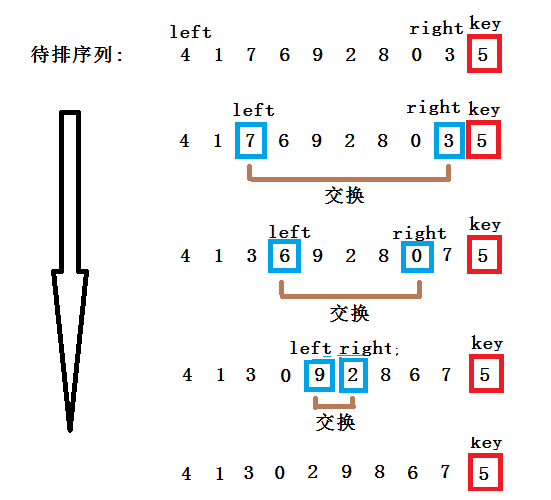当left >= right时，一趟快速排序就完成了，这时将Key和array[left]的值进行一次交换。 一次快排的结果:4 1 3 0 2 5 8 6 7 9
基于这种思想，可以写出代码：
int PartSort(int* array,int left,int right)
{
int& key = array[right];
while(left < right)
{
while(left < right && array[left] <= key)
{
++left;
}
while(left < right && array[right] >= key)
{
--right;
}
swap(array[left],array[right]);
}
swap(array[left],key);
return left;
}

问题：下面的代码为什么还要判断left < right？
while(left < right && array[left] <= key)

key是整段序列最后一个，right是key前一个位置，如果array[right]这个位置的值和key相等，满足array[left] <= key，然后++left,这时候left会走到key的下标处。
###挖坑法
选取一个关键字(key)作为枢轴，一般取整组记录的第一个数/最后一个，这里采用选取序列最后一个数为枢轴，也是初始的坑位。设置两个变量left = 0;right = N - 1;从left一直向后走，直到找到一个大于key的值，然后将该数放入坑中，坑位变成了array[left]。right一直向前走，直到找到一个小于key的值，然后将该数放入坑中，坑位变成了array[right]。重复3和4的步骤，直到left和right相遇，然后将key放入最后一个坑位。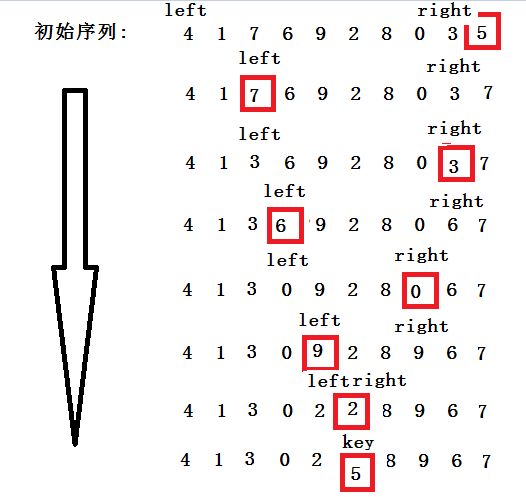当left >= right时，将key放入最后一个坑，就完成了一次排序。 注意，left走的时候right是不动的，反之亦然。因为left先走，所有最后一个坑肯定在array[right]。
写出代码：
int PartSort(int* array,int left,int right)
{
int key = array[right];
while(left < right)
{
while(left < right && array[left] <= key)
{
++left;
}
array[right] = array[left];
while(left < right && array[right] >= key)
{
--right;
}
array[left] = array[right];
}
array[right] = key;
return right;
}

###前后指针法
定义变量cur指向序列的开头，定义变量pre指向cur的前一个位置。当array[cur] < key时，cur和pre同时往后走，如果array[cur]>key，cur往后走，pre留在大于key的数值前一个位置。当array[cur]再次 < key时，交换array[cur]和array[pre]。
通俗一点就是，在没找到大于key值前，pre永远紧跟cur，遇到大的两者之间机会拉开差距，中间差的肯定是连续的大于key的值，当再次遇到小于key的值时，交换两个下标对应的值就好了。
带着这种思想，看着图示应该就能理解了。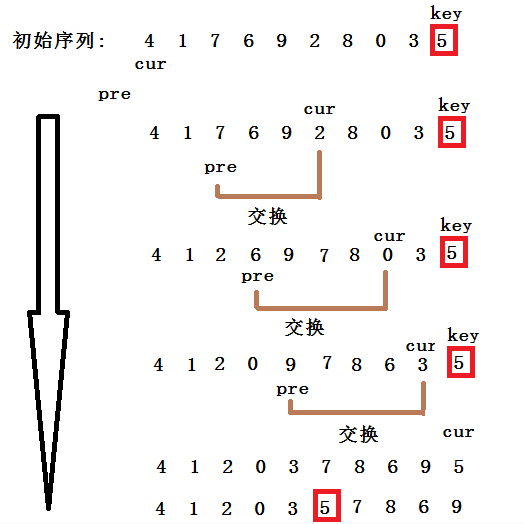下面是实现代码：
int PartSort(int* array,int left,int right)
{
if(left < right){
int key = array[right];
int cur = left;
int pre = cur - 1;
while(cur < right)
{
while(array[cur] < key && ++pre != cur)//如果找到小于key的值，并且cur和pre之间有距离时则进行交换。注意两个条件的先后位置不能更换，可以参照评论中的解释
{
swap(array[cur],array[pre]);
}
++cur;
}
swap(array[++pre],array[right]);
return pre;
}
return -1;
}

最后的前后指针法思路有点绕，多思考一下就好了。它最大的特点就是，左右指针法和挖坑法只能针对顺序序列进行排序，如果是对一个链表进行排序， 就无用武之地了。
所以记住了，前后指针这个特点！

###快速排序的优化
首先快排的思想是找一个枢轴，然后以枢轴为中介线，一遍都小于它，另一边都大于它，然后对两段区间继续划分，那么枢轴的选取就很关键。
1、三数取中法 上面的代码思想都是直接拿序列的最后一个值作为枢轴，如果最后这个值刚好是整段序列最大或者最小的值，那么这次划分就是没意义的。 所以当序列是正序或者逆序时，每次选到的枢轴都是没有起到划分的作用。快排的效率会极速退化。
所以可以每次在选枢轴时，在序列的第一，中间，最后三个值里面选一个中间值出来作为枢轴，保证每次划分接近均等。
2、直接插入 由于是递归程序，每一次递归都要开辟栈帧，当递归到序列里的值不是很多时，我们可以采用直接插入排序来完成，从而避免这些栈帧的消耗。
整个代码：
//三数取中
int GetMid(int* array,int left,int right)
{
assert(array);
int mid = left + ((right - left)>>1);
if(array[left] <= array[right])
{
if(array[mid] <  array[left])
return left;
else if(array[mid] > array[right])
return right;
else
return mid;
}
else
{
if(array[mid] < array[right])
return right;
else if(array[mid] > array[left])
return left;
else
return mid;
}

}

//左右指针法
int PartSort1(int* array,int left,int right)
{
assert(array);
int mid = GetMid(array,left,right);
swap(array[mid],array[right]);

int& key = array[right];
while(left < right)
{
while(left < right && array[left] <= key)//因为有可能有相同的值，防止越界，所以加上left < right
++left;
while(left < right && array[right] >= key)
--right;

swap(array[left],array[right]);
}

swap(array[left],key);
return left;
}

//挖坑法
int PartSort2(int* array,int left,int right)
{
assert(array);
int mid = GetMid(array,left,right);
swap(array[mid],array[right]);

int key = array[right];
while(left < right)
{
while(left < right && array[left] <= key)
++left;
array[right] = array[left];

while(left < right && array[right] >= key)
--right;
array[left] = array[right];
}
array[right] = key;
return right;
}

//前后指针法
int PartSort3(int* array,int left,int right)
{
assert(array);
int mid = GetMid(array,left,right);
swap(array[mid],array[right]);
if(left < right){
int key = array[right];
int cur = left;
int pre = left - 1;
while(cur < right)
{
while(array[cur] < key && ++pre != cur)
{
swap(array[cur],array[pre]);
}
++cur;
}
swap(array[++pre],array[right]);
return pre;
}
return -1;
}

void QuickSort(int* array,int left,int right)
{
assert(array);
if(left >= right)
return;

//当序列较短时，采用直接插入
if((right - left) <= 5)
InsertSort(array,right-left+1);

int index = PartSort3(array,left,right);
QuickSort(array,left,index-1);
QuickSort(array,index+1,right);
}

int main()
{
int array[] = {4,1,7,6,9,2,8,0,3,5};
QuickSort(array,0,sizeof(array)/sizeof(array) -1);//因为传的是区间，所以这里要 - 1；
}


非递归实现
递归的算法主要是在划分子区间，如果要非递归实现快排，只要使用一个栈来保存区间就可以了。 一般将递归程序改成非递归首先想到的就是使用栈，因为递归本身就是一个压栈的过程。
void QuickSortNotR(int* array,int left,int right)
{
assert(array);
stack<int> s;
s.push(left);
s.push(right);//后入的right，所以要先拿right
while(!s.empty)//栈不为空
{
int right = s.top();
s.pop();
int left = s.top();
s.pop();

int index = PartSort(array,left,right);
if((index - 1) > left)//左子序列
{
s.push(left);
s.push(index - 1);
}
if((index + 1) < right)//右子序列
{
s.push(index + 1);
s.push(right);
}
}
}

上面就是关于快速排序的一些知识点，如果哪里有错误，还望指出。
展开全文快速排序 递归
• 汉诺塔（Hanoi） 算法Java实现。通过三个函数，分别对Hanoi进行递归、非递归非递归规律实现。
• 包含二叉树的递归建立，非递归建立，先序非递归遍历，后序非递归遍历，C代码，是在TC环境下完全调试好的，并在文档中给予了详细的使用方法--LZL
• 包含一下方法: 1.通过一个数组来构造一颗...6.使用非递归 先序遍历一棵二叉树 7.使用非递归 中序遍历一棵二叉树 8.使用非递归 后序遍历一棵二叉树 PS:代码为C++代码 可以直接下载使用！！！ PS2:每句代码都有详细注释
• 非递归源码，希望可以帮助大家了解一下树非递归的思想，谢谢。
• 由于递归创建二叉树的效率太低，所以我们需要采取非递归的方式来创建和访问一颗二叉树。因为栈和递归的原理相同，所以任何递归方法都可以借助栈转化成非递归的方法。下面我们使用非递归的方式 ，采用先序创建来创建...
       由于递归创建二叉树的效率太低，所以我们需要采取非递归的方式来创建和访问一颗二叉树。因为栈和递归的原理相同，所以任何递归方法都可以借助栈转化成非递归的方法。下面我们使用非递归的方式 ，采用先序创建来创建一颗二叉树。并且提供前、中、后三种非递归的遍历方式。

在这里我们要借助一个flag来标记所需要的查询，flag=1表示创建左子树，flag=2表示创建右子树，flag=3就要将已经创建好的节点出栈。非递归访问也需要借助flag来完成。

程序的执行流程如下图：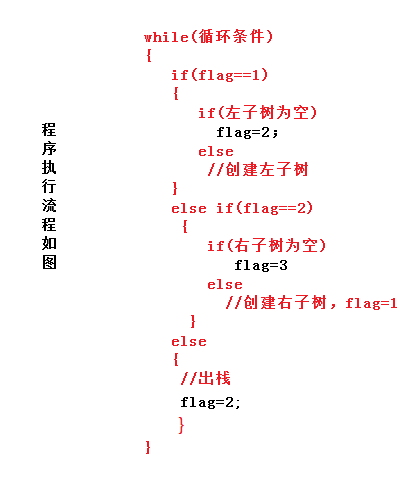#pragma once
#include<cassert>
#include<stack>
using namespace std;

template<typename T>
struct TreeNode
{
T _data;
TreeNode *_left;
TreeNode *_right;
TreeNode(const T& data=T())
:_data(data)
, _left(NULL)
,_right(NULL)
{}
};

template<typename T>
class Tree
{
typedef TreeNode<T> Node;
public:
Tree()
:_root(NULL){}
Tree(const T* a, size_t size, const T& invalid)
{
size_t index = 0;
_root=_CreatTree(a,size,index,invalid);
}

void PrevOder()
{
assert(_root);
Node *root = _root;

stack<Node*> s;
s.push(root);
int flag = 1;

Node *cur = NULL;
while (!s.empty())
{
cur = s.top();
if (flag == 1)                  //遍历左子树
{
if (cur->_left == NULL)    //如果左子树为空，则flag=2
{
flag = 2;
}
else
{
cout << cur->_data << " ";    //先序输出，先输出根结点
s.push(cur->_left);
}
}
else if (flag == 2)
{
if (cur->_right == NULL)
{
flag = 3;
}
else
{
s.push(cur->_right);
flag = 1;
}
}
else
{
Node *tmp = NULL;
if (!s.empty())
{
tmp = s.top();
s.pop();
}
cur = tmp;
while (!s.empty() && s.top()->_right == tmp)  //将输出过的结点出栈
{
tmp = s.top();
s.pop();
}
if (!s.empty())         //输出右子树的叶子节点
{
cout << cur->_data << " ";
}
flag = 2;
}
}
cout << endl;
}

void InOder()
{
assert(_root);
Node *root =_root;
stack<Node*> s;
s.push(root);
int flag = 1;
while (!s.empty())
{
Node *cur = s.top();
if (flag == 1)                  //先遍历左子树
{
if (cur->_left == NULL)
{
flag = 2;
}
else
{
s.push(cur->_left);
}
}
else if (flag == 2)        //遍历右子树
{
if (cur->_right == NULL)
{
flag = 3;
}
else
{
cout << cur->_data << " ";        //输出右子树的跟结点
s.push(cur->_right);
flag = 1;
}
}
else
{
Node* tmp = NULL;
if (!s.empty())
{
tmp = s.top();
s.pop();
}
cout << tmp->_data << " ";          //输出左子树结点和左子树的根结点

while (!s.empty() && s.top()->_right == tmp)
{
tmp = s.top();
s.pop();
}
flag = 2;
}
}
cout << endl;
}

void PostOder()
{
assert(_root);
Node *root = _root;
stack<Node*> s;

int flag = 1;       //=1遍历左子树，=2 遍历右子树，=3 输出结点
s.push(root);       //根节点入栈
Node *cur = NULL;

while (!s.empty())
{
cur = s.top();
if (flag == 1)              //遍历左子树
{
if (cur->_left== NULL)   //如果左子树为空，则flag=2,准备遍历右子树
{
flag = 2;
}
else
{
s.push(cur->_left);
}
}
else if (flag == 2)             //遍历右子树
{
if (cur->_right == NULL)       //右子树为空，准备输出结点
{
flag = 3;
}
else
{
s.push(cur->_right);
flag = 1;                //遍历当前结点的左子树
}
}
else
{
Node *tmp = NULL;
if (!s.empty())
{
tmp = s.top();    //保存当前栈顶元素
s.pop();          //抛出栈顶元素
}
cout << tmp->_data << " ";    //输出结点的信息

//如果当前栈顶元素的右子树是tmp，则抛出tmp之后按照后序也要抛出栈顶元素
while (!s.empty() && s.top()->_right == tmp)
{
tmp = s.top();    //保存当前栈顶元素
s.pop();          //抛出栈顶元素
cout << tmp->_data << " ";    //输出结点的信息
}
flag = 2;
}
}
cout << endl;
}

protected:
//非递归先序创建二叉树
Node *_CreatTree(const T* a,size_t size,size_t index,const T& invalid)
{
stack<Node*> s;
Node* root = new Node(a[index++]);
int flag = 1;         //flag  =1创建左孩子，=2创建右孩子，=3出栈
s.push(root);
Node *tmp = NULL;
Node *cur = NULL;
while (index<size)
{
if (flag == 1)      //创建左孩子
{
if (a[index] == invalid)     //如果左孩子为空，则flag=2创建右孩子
{
flag = 2;
}
else
{
cur = new Node(a[index]);
tmp = s.top();
tmp->_left = cur;          //链接到左指针上
s.push(cur);               //将新节点压入栈中
}
}
else if (flag == 2)              //flag=2,创建右孩子
{
if (a[index] == invalid)        //如果右孩子为空，则flag=3准备出栈
{
flag = 3;
}
else
{
cur = new Node(a[index]);
tmp = s.top();
tmp->_right= cur;          //链接到左指针上
s.push(cur);               //将新节点压入栈中
flag = 1;                  //再创建新结点的左孩子
}
}
else
{
if (!s.empty())
{
tmp = s.top();
s.pop();
}
//如果已经出栈的元素是当前栈顶的右孩子，则表示这个结点的左右子树已经创建完毕，则一直出栈
while (!s.empty() && s.top()->_right == tmp)
{
tmp = s.top();
s.pop();
}

flag = 2;
index--;
}
index++;
}
return root;
}

protected:
Node *_root;
};

//测试

#include<iostream>
#include"Tree.h"
using namespace std;

void test()
{
int arr[] = {1,2,3,'#','#',4,'#','#',5,6};
size_t  sz = sizeof(arr) / sizeof(arr);
Tree<int> t(arr,sz,'#');
t.PostOder();
t.PrevOder();
t.InOder();
}

int main()
{
test();
system("pause");
return 0;
}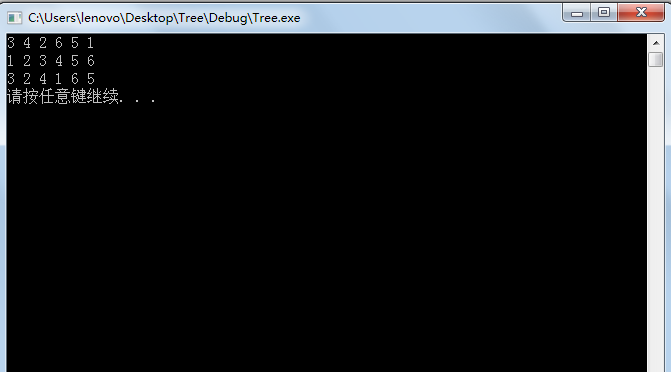展开全文• 非递归查找的简单C语言程序，供初学者参考一下，哈哈。
• 八皇后递归及非递归实现源码； 八皇后递归及非递归实现源码
• 快速排序算法设计与分析总结 二叉树与树的转换前序、后序的递归、非递归算法，层次序的非递归算法的实现 二叉树与树的转换前序、后序的递归、非递归算法，层次序的非递归算法的实现，应包含建树的实现 实现树与...
• 易语言非递归算法遍历目录源码,非递归算法遍历目录,枚举文件
• 主要介绍了数据结构 二叉树的递归与非递归的相关资料,需要的朋友可以参考下
• 快速排序算法设计与分析总结 二叉树与树的转换前序、后序的递归、非递归算法，层次序的非递归算法的实现 实现树与二叉树的转换的实现。以及树的前序、后序的递归、非递归算法，层次序的非递归算法的实现，应包含建树...快速排序算法
• 易语言源码非递归算法遍历目录.rar 易语言源码非递归算法遍历目录.rar 易语言源码非递归算法遍历目录.rar 易语言源码非递归算法遍历目录.rar 易语言源码非递归算法遍历目录.rar 易语言源码非递归算法遍历目录....
• 八皇后的非递归代码：全部代码分享，方便各位java初学者学习
• ## 详解二叉树的非递归遍历

万次阅读 多人点赞 2018-06-29 11:18:20
前言 　对于二叉树的递归遍历比较简单，所以本文主要讨论的是非递归版。其中，中序遍历的非递归写法最简单，后序遍历最难。 节点的定义： //Binary Tree Node typedef struct node { int data; ...
• 数据结构实验二叉树用递归实现先序遍历、中序遍历和后序遍历，用几种不同非递归方法实现了中序遍历，代码附有详细注释
•数据结构
• ## 递归和非递归

千次阅读 2019-04-13 01:38:26
2、非递归就是不断地对参数入栈、出栈，省去了函数层层展开、层层调用的开销。虽然参数出入栈次数多了，但是一般都开辟固定的足够大的内存来一次性开辟、重复使用。 3、非递归是从堆栈的角度来编写程序，速度快，但...
• 主要为大家详细介绍了C语言非递归后序遍历二叉树，具有一定的参考价值，感兴趣的小伙伴们可以参考一下
• 此程序基于清华大学出版社一书中的非递归迷宫求解问题，在迷宫生成中运用了深度优先和随机生成种子的方法，在迷宫寻路中运用了非递归的追溯法，并且添加了开场动画。
• 主要介绍了C++非递归建立二叉树的方法,实例分析了二叉树的原理与C++实现技巧,具有一定参考借鉴价值,需要的朋友可以参考下
• 主要介绍了C++ 中二分查找递归非递归实现并分析的相关资料,需要的朋友可以参考下
• 主要介绍了C++基于递归和非递归算法求二叉树镜像的方法,针对二叉树遍历结合实例形式分析了递归与非递归算法的实现与使用技巧,需要的朋友可以参考下...